### Home > MC2 > Chapter 10 > Lesson 10.1.6 > Problem10-80

10-80.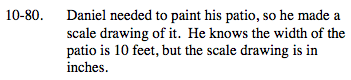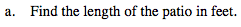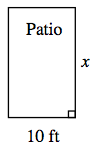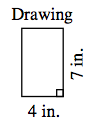Find the ratio between two corresponding sides and use it to create a proportion or to multiply as a scale factor and find the missing length.

x = 17.5 feet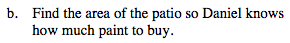The area ratio is the (scale factor)². Multiply the area ratio by the scale drawing area to get the actual area of the patio.

Patio Area: 175 square feet

$\text{If the scale factor from drawing to patio is \frac{5}{2},} \text{ what would the area ratio be?}$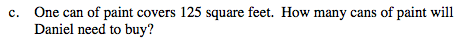If each can of paint covers 125 square feet, how many cans are needed to cover 175 square feet?

Daniel is only able to buy full cans of paint. Round up the cans of paint if it does not come out to an even full number.